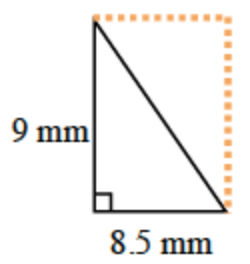### Home > CC1 > Chapter 5 > Lesson 5.3.3 > Problem5-92

5-92.

Find the area of the following triangles. Show all your work.

1.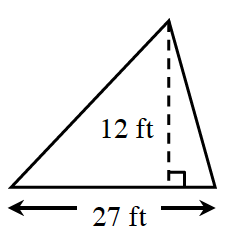Two of these triangles make a parallelogram, what is the area of the parallelogram?

To find the area of a parallelogram, multiply the base by the height. What does the area of the triangle have to be?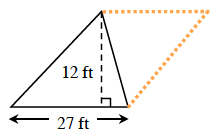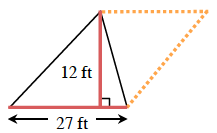1.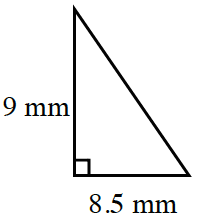What is the area of the rectangle at right? Use it to find the area of the original triangle.